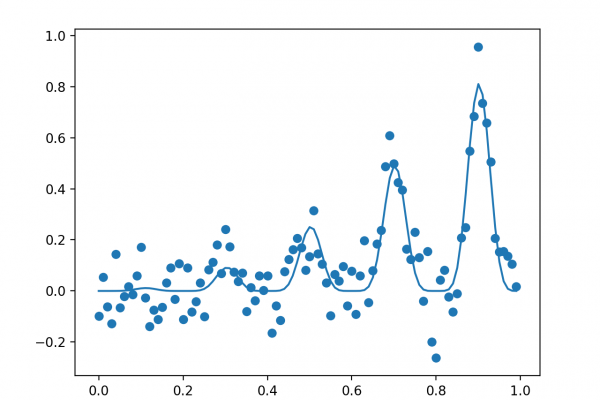# How to Implement Bayesian Optimization from Scratch in PythonLast Updated on August 22, 2020

In this tutorial, you will discover how to implement the Bayesian Optimization algorithm for complex optimization problems.

Global optimization is a challenging problem of finding an input that results in the minimum or maximum cost of a given objective function.

Typically, the form of the objective function is complex and intractable to analyze and is often non-convex, nonlinear, high dimension, noisy, and computationally expensive to evaluate.

Bayesian Optimization provides a principled technique based on Bayes Theorem to direct a search of a global optimization problem that is efficient and effective. It works by building a probabilistic model of the objective function, called the surrogate function, that is then searched efficiently with an acquisition function before candidate samples are chosen for evaluation on the real objective function.

Bayesian Optimization is often used in applied machine learning to tune the hyperparameters of a given well-performing model on a validation dataset.

After completing this tutorial, you will know:

• Global optimization is a challenging problem that involves black box and often non-convex, non-linear, noisy, and computationally expensive objective functions.
• Bayesian Optimization provides a probabilistically principled method for global optimization.
• How to implement Bayesian Optimization from scratch